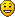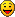## MoBot 2

Posts: 266

Joined: Mon Mar 08, 2010 5:34 pm

Location: Mesa AZ | Ithaca NY

### MoBot 2

My friend Buvet mentioned that it might be cool to play a bitfighter, league of legends cross over, so I've been working on one. It's not as good as it could be but I think it's pretty good so far.

This bot is level specific, so please get the map:
http://bitfighter.org/levels/levels/view/418

I find that the more bots, the better.
It seems almost impossible to make bots push-overs for players (like they are supposed to be in LoL), so I made them completely ignore normal ships and only kill bots and cores.

Tell me what you thinkCode:
`--------------------------------------------------------------------------------- This bot is adapted from Thread's Mobot code, which was adapted from the S_bot.-- S_bots' authors deserve their due credit, as does Thread (obviously).-- Special thanks to Fordcars---- Any original coding beyond that:--  Furbuggy-------------------------------------------------------------------------------goalPt = point.new(0,0);prevtarget = nil;gotoPositionWasNil = true;o = point.new(0,0);botPos = nil;items = { };--BOTA specific variables--First, the different paths the bot can take.top1 = {point.new(510,-5100), point.new(892.5,-6375.0), point.new(1875,-7375), point.new(3315.0,-7905.0), point.new(3901,-7880.0), point.new(5610.0,-7884.0), point.new(7395.0,-7267.5)};mid1 = {point.new(2550.0, -2295.0), point.new(4590.0, -4080.0), point.new(4796, -4633.0), point.new(5355.0, -5227.5), point.new(6177.5, -6015), point.new(7395.0, -7267.5)};bot1 = {point.new(6120,-382.5), point.new(7140.0,-1020.5), point.new(7640,-1869.5), point.new(7983.0,-3736.0), point.new(8046.9,-5527.1), point.new(7395,-7267.5)};top2 = {point.new(2550, -7777.0), point.new(650, -6258), point.new(308, -4392), point.new(24,-2601), point.new(637,-637)};mid2 = {point.new(5865.0, -6120.0), point.new(3570.0,-3825.0), point.new(2926, 2926), point.new(2113, -2113), point.new(637,-637)}; bot2 = {point.new(7905,-2550), point.new(6375,-765), point.new(5355,-255), point.new(4206,-255), point.new(2550,-255), point.new(637,-637)};myPath = {};soFar = 0;pathSize = 8;-- This function gets run once during setup.  It is the only time we can declare variables-- and have them be defined as globals.function main()    botRadius = bot:getRad();    dirToGo = 0;    game = bf:getGameInfo();    difficulty         = tonumber(arg) or .1;    agression          = tonumber(arg) or 0.5;    defense            = tonumber(arg) or 0;    speed              = tonumber(arg) or .65;       directionThreshold = tonumber(arg) or .25;    gameType = game:getGameType();        averageIndex = 1;    averageMax = 20;    averageArray = { };    for i = 1, averageMax do        averageArray[i] = false;    end    myOrbitalDirection = 1;end-- bot:fireModule(Module.Shield);function angleDifference(angleA, angleB)    return (math.mod(math.mod(angleA - angleB, math.pi * 2) + math.pi * 3, math.pi * 2) - math.pi)endfunction fireAtObjects()   result = false;    table.clear(items);    bot:findVisibleObjects(items, ObjType.Robot, ObjType.Turret, ObjType.Core);    -- Cycle through list of potential items until we find one that we can attack    for index, enemy in ipairs(items) do        if(fireAtObject(enemy, Weapon.Phaser)) then         result = true;            break;        end    end      return result;end-- Fires at the specified object with the specified weapon if the obj is a good target. Returns whether it fired or not.-- This is more than I need for my purposes, but it covers all the cases I need, so I will leave it as is.function fireAtObject(obj, weapon)    local classId = obj:getObjType()    if(classId == ObjType.Turret or classId == ObjType.ForceFieldProjector) then        --Ignore all same-team engineered objects and essentially dead objects        if (obj:getTeamIndex() == bot:getTeamIndex() or obj:getHealth() < .1) then            return false;        end    end    --No shooting various team related objects    if (obj:getTeamIndex() == bot:getTeamIndex() and game:isTeamGame()) or        obj:getTeamIndex() == Team.Neutral then        if (classId == ObjType.Ship or classId == ObjType.Robot or            classId == ObjType.Core or classId == ObjType.SpyBug) then            return false;        end    end    --No shooting non-flag carriers in single player rabbit    if gameType == GameType.Rabbit and not game:isTeamGame() and         (classId == ObjType.Ship or classId == ObjType.Robot) and         not bot:hasFlag() and not obj:hasFlag() then        return false;    end    --We made it here!  We have a valid target..    local angle = getFiringSolution(obj);    if angle ~= nil and bot:hasWeapon(weapon) then        bot:setAngle(angle + math.rad((math.random()-0.5)*20*(1-difficulty)));        bot:fireWeapon(weapon);        return(true);    end    return(false)endfunction getName()     return("MOBOT");endfunction gotoPosition(pt)    if pt ~= nil then        gotoPositionWasNil = false;        goalPt = bot:getWaypoint(pt);      if(goalPt ~= nil) then         dirToGo = point.angleTo(botPos, goalPt);        end    endend -- Returns the objective for the bot, in the form of a point the bot can navigate towards.function getObjective()   local result = nil;      local dest = myPath[soFar];   gotoPosition(dest);      --Move to next waypoint?   if (point.distSquared(bot:getPos(), dest) <= 250 and soFar < pathSize) then      soFar = soFar + 1;   end       return result;endfunction doObjective()   --get destination object   if (soFar ~= 0) then      local obj = getObjective();      if obj ~= nil then         gotoPosition(obj:getPos());      end   endendfunction goInDirection()    bot:setThrust(speed, dirToGo);endfunction onTick(deltaTime)   -- Since team hasn't been assigned when main runs, I have to do my checking in onTick   -- Set path if soFor is still 0   if (bot:getHealth () == 0) thenmyPath = {};soFar= 0;returnend   if (soFar == 0) then      if (bot:getTeamIndex() > 0) then             if (bot:getTeamIndex() == 1) then             if (bot:getPos().y < -1530) then               myPath = top1;                 elseif (bot:getPos().y > -800) then               myPath = bot1;              else             myPath = mid1;            end             elseif (bot:getTeamIndex() == 2) then              if (bot:getPos().y < -7400) then               myPath = top2;                elseif (bot:getPos().y > -6700) then               myPath = bot2;             else             myPath = mid2;            end         end         soFar = 1;      end   end       botPos = bot:getPos();    assert(bot:getPos() ~= nil);       if (not fireAtObjects()) then      doObjective();      goInDirection();   endend`
BAM! I'm back.Posts: 985

Joined: Fri Apr 20, 2012 3:51 pm

Location: Some city, somewhere

### Re: MoBot 2

Awesome! Much League of Legends!
skybax: Why is this health pack following me?
bobdaduck: uh, it likes you.

Posts: 1319

Joined: Thu Apr 19, 2012 2:57 pm

### Re: MoBot 2

Not Bas maybe you can make s_bot smarter for us.Good level to preety wel desinged.
Bitfighter Forever.